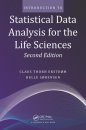United States
£ GBP
EU Shipping Update - read more

## British Wildlife

8 issues per year 84 pages per issue Subscription only

British Wildlife is the leading natural history magazine in the UK, providing essential reading for both enthusiast and professional naturalists and wildlife conservationists. Published eight times a year, British Wildlife bridges the gap between popular writing and scientific literature through a combination of long-form articles, regular columns and reports, book reviews and letters.

Subscriptions from £40 per year

## Conservation Land Management

4 issues per year 44 pages per issue Subscription only

Conservation Land Management (CLM) is a quarterly magazine that is widely regarded as essential reading for all who are involved in land management for nature conservation, across the British Isles. CLM includes long-form articles, events listings, publication reviews, new product information and updates, reports of conferences and letters.

Subscriptions from £22 per year

# Introduction to Statistical Data Analysis for the Life Sciences

Textbook
By: Claus Thorn Ekstrøm(Author), Helle Sørensen(Author)
514 pages, 101 b/w illustrations, 97 tables
Click to have a closer look
•ISBN: 9781482238938 Edition: 2 Paperback Dec 2014 Usually dispatched within 1-2 weeks
£54.99
#216568

Expanded with over 100 more pages, Introduction to Statistical Data Analysis for the Life Sciences, Second Edition presents the right balance of data examples, statistical theory, and computing to teach introductory statistics to students in the life sciences. This popular textbook covers the mathematics underlying classical statistical analysis, the modelling aspects of statistical analysis and the biological interpretation of results, and the application of statistical software in analysing real-world problems and datasets.

New to the Second Edition
- A new chapter on non-linear regression models
- A new chapter that contains examples of complete data analyses, illustrating how a full-fledged statistical analysis is undertaken
- Additional exercises in most chapters
- A summary of statistical formulas related to the specific designs used to teach the statistical concepts

This text provides a computational toolbox that enables students to analyse real datasets and gain the confidence and skills to undertake more sophisticated analyses. Although accessible with any statistical software, the text encourages a reliance on R. For those new to R, an introduction to the software is available in an appendix. Introduction to Statistical Data Analysis for the Life Sciences also includes end-of-chapter exercises as well as an entire chapter of case exercises that help students apply their knowledge to larger datasets and learn more about approaches specific to the life sciences.

## Contents

Description of Samples and Populations
- Data types
- Visualizing categorical data
- Visualizing quantitative data
- Statistical summaries
- What is a probability?
- R

Linear Regression
- Fitting a regression line
- When is linear regression appropriate?
- The correlation coefficient
- Perspective
- R

Comparison of Groups
- Graphical and simple numerical comparison
- Between-group variation and within-group variation
- Populations, samples, and expected values
- Least squares estimation and residuals
- Paired and unpaired samples
- Perspective
- R

The Normal Distribution
- Properties
- One sample
- Are the data (approximately) normally distributed?
- The central limit theorem
- R

Statistical Models, Estimation, and Confidence Intervals
- Statistical models
- Estimation
- Confidence intervals
- Unpaired samples with different standard deviations
- R

Hypothesis Tests
- Null hypotheses
- t-tests
- Tests in a one-way ANOVA
- Hypothesis tests as comparison of nested models
- Type I and type II errors
- R

Model Validation and Prediction
- Model validation
- Prediction
- R

Linear Normal Models
- Multiple linear regression
- Additive two-way analysis of variance
- Linear models
- Interactions between variables
- R

Non-Linear Regression
- Non-linear regression models
- Estimation, confidence intervals, and hypothesis tests
- Model validation
- R

Probabilities
- Outcomes, events, and probabilities
- Conditional probabilities
- Independence

The Binomial Distribution
- The independent trials model
- The binomial distribution
- Estimation, confidence intervals, and hypothesis tests
- Differences between proportions
- R

Analysis of Count Data
- The chi-square test for goodness-of-fit
- 2 x 2 contingency table
- Two-sided contingency tables
- R

Logistic Regression
- Odds and odds ratios
- Logistic regression models
- Estimation and confidence intervals
- Hypothesis tests
- Model validation and prediction
- R

Statistical Analysis Examples
- Water temperature and frequency of electric signals from electric eels
- Association between listeria growth and RIP2 protein
- Effect of an inhibitor on the chemical reaction rate
- Birthday bulge on the Danish soccer team
- Animal welfare
- Monitoring herbicide efficacy

Case Exercises
- Case 1: Linear modeling
- Case 2: Data transformations
- Case 3: Two sample comparisons
- Case 4: Linear regression with and without intercept
- Case 5: Analysis of variance and test for linear trend
- Case 6: Regression modeling and transformations
- Case 7: Linear models
- Case 8: Binary variables
- Case 9: Agreement
- Case 10: Logistic regression
- Case 11: Non-linear regression
- Case 12: Power and sample size calculations

Appendix A: Summary of Inference Methods
Appendix B: Introduction to R
Appendix C: Statistical Tables
Appendix D: List of Examples Used throughout the Book

Bibliography
Index

## Customer Reviews

Textbook
By: Claus Thorn Ekstrøm(Author), Helle Sørensen(Author)
514 pages, 101 b/w illustrations, 97 tables